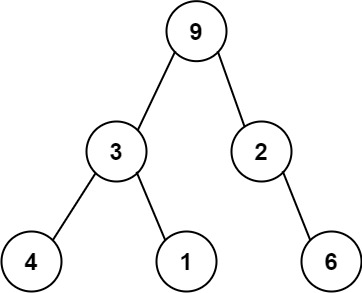GeetCode Hub

One way to serialize a binary tree is to use preorder traversal. When we encounter a non-null node, we record the node's value. If it is a null node, we record using a sentinel value such as '#'.For example, the above binary tree can be serialized to the string "9,3,4,#,#,1,#,#,2,#,6,#,#", where '#' represents a null node.

Given a string of comma-separated values preorder, return true if it is a correct preorder traversal serialization of a binary tree.

It is guaranteed that each comma-separated value in the string must be either an integer or a character '#' representing null pointer.

You may assume that the input format is always valid.

• For example, it could never contain two consecutive commas, such as "1,,3".

Note: You are not allowed to reconstruct the tree.

Example 1:

Input: preorder = "9,3,4,#,#,1,#,#,2,#,6,#,#"
Output: true

Example 2:

Input: preorder = "1,#"
Output: false

Example 3:

Input: preorder = "9,#,#,1"
Output: false

Constraints:

• 1 <= preorder.length <= 104
• preoder consist of integers in the range [0, 100] and '#' separated by commas ','.

class Solution { public boolean isValidSerialization(String preorder) { } }